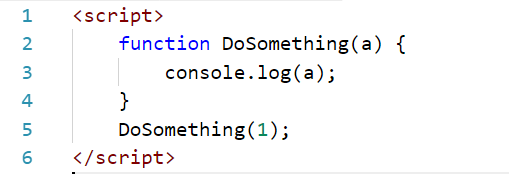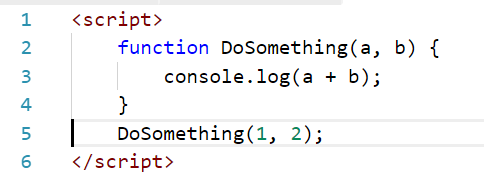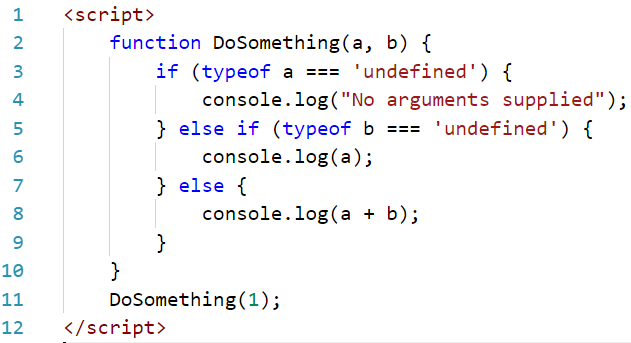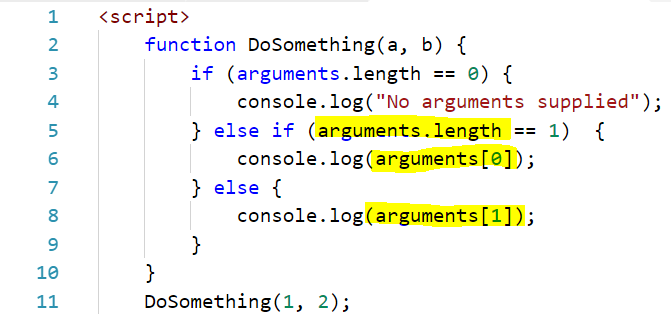Function Overloading is the ability to define two or more functions with the same name that have a different signature, i.e. a number and/or different types of parameters/arguments.

For example:

function DoSomething(a)

function DoSomething(a,b)

function DoSomething(a,b,c)

In JavaScript, there is no function overloading. There is however behavior regarding sending different parameters, and there is a pattern we can use to handle function overloading.

First, consider the case above. If we have one argument, we can call a simple function like:Which writes 1 to the console:Now if we expand this to 2 arguments, we can add both:This writes 3 to the console:Now what if we didn’t supply the 2nd argument? We can check for this by:JavaScript also defines an arguments object. You can access arguments variables through:Another way is to use an object as a parameter, which can hold many arguments. For example:

```    function DoSomething(o) {
if (o["arg1"])
console.log(o["arg1"]);
}
DoSomething({"arg1":"Test", "arg2":"Test2"});
```

This produces: